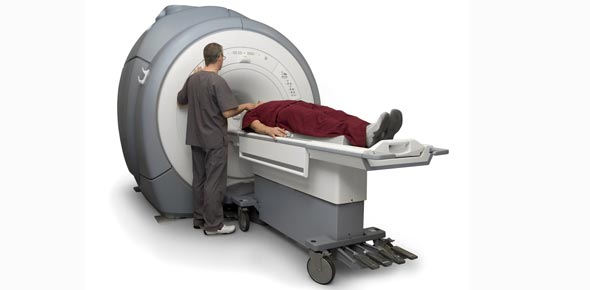# Difference Between T1 And T2 Weighted MRI!

11 QuestionsSettingsThe magnetic resonance imagining is the current advanced technology that doctors use to take pictures of the internal human body. Generally, there are two types of weighted MRI procedures that are T1 and T2. Find out their differences below.

Related Topics
• 1.
The lymph nodes of the neck can be classified or divided into how many levels or regions for ease of identification?
• A.

5

• B.

6

• C.

7

• D.

8

• 2.
Questions 49 through 52 refer to the figure below of an axial, T1-weighted MRI of the sublingual glands.Which arrow points to the genioglossus muscle?
• A.

A

• B.

B

• C.

C

• D.

D

• 3.
Questions 53 through 57 refer to the figure below of an axial, T1-weighted MRI of the neck.Which arrow points to the levator scapulae muscle?
• A.

A

• B.

B

• C.

C

• D.

D

• E.

E

• 4.
Questions 53 through 57 refer to the figure below of an axial, T1-weighted MRI of the neck.Which arrow points to the middle scalene muscle?
• A.

A

• B.

B

• C.

C

• D.

D

• E.

E

• 5.
Questions 59 through 63 refer to the figure below of an axial CT of the brain.Which arrow points to the basilar artery in the prepontine cistern?
• A.

A

• B.

B

• C.

C

• D.

D

• E.

E

• 6.
Questions 20 through 23 refer to the figure below of an axial, T2-weighted MRI of the lumbar vertebra.Which arrow points to the thecal sac?
• A.

A

• B.

B

• C.

C

• D.

D

• 7.
Questions 44 through 48 refer to the figure below of a coronal CT reformat of the neck.Which arrow points to the epiglottis?
• A.

A

• B.

B

• C.

C

• D.

D

• E.

E

• 8.
• A.

A

• B.

B

• C.

C

• D.

D

• E.

E

• 9.
Questions 44 through 48 refer to the figure below of a coronal CT reformat of the neck.Which arrow points tot he thyroid gland?
• A.

A

• B.

B

• C.

C

• D.

D

• E.

E

• 10.
Questions 36 through 39 refer to the figure below of a sagittal CT reformat of the pharynx.Which arrow points to the hard palate?
• A.

A

• B.

B

• C.

C

• D.

D